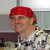Ms Excel QuestionTags:
Microsoft Excel
Microsoft Excel formulas
i have entered different students name in column A, Paper name in Column B & their marks in column C. like: john english 90 magi maths 15 john stats 99 john history 35 & i've applied this formula: =if(A2:A66000="John",sum(C2:C66000)) to get the total marks of John in all papers,but it doesnt work. plz help...!!!

Thanks. We'll let you know when a new response is added.

Funny you should ask. I had to figure it out today myself.

I would use the following formula for your issue:

=SUMPRODUCT((A2:A66000=”John”)*((C2:C66000)*1))

However, I wonder if you want the average rather than the sum. If so, use this formula:

=SUMPRODUCT((A1:A66000=”John”)*((C1:C66000)*1))/SUMPRODUCT((A1:A66000=”John”)*1)

The following is what I did today which used named ranges (I find it easier to read).

I created named ranges. For example: Tax Type is C6:C39 and EnvTotal is D6:D39. I then Multiplied the sum (SUMPRODUCT) by RegularTax which is E2 to get the total tax for the items that were taxed at the regular tax value (“R”).

=SUMPRODUCT((TaxType=”R”)*(EnvTotal*1))*RegularTax

I hope this helps.

Tammy

the answer to your query is really simple:
1 name sub marks
2 john english 90
3 maggi maths 15
4 John stats 99
5 john history 35

total marks of john:
formula: =sumif(A2:A5,”john”,C2:C5)

Discuss This Question: 1 Reply

There was an error processing your information. Please try again later.
Thanks. We'll let you know when a new response is added.
Send me notifications when members answer or reply to this question.
•Here's a simpler solution that doesn't require hard-coding students' names. Use the SUBTOTAL() function for your formula(s), and click the AutoFilter button. SUBTOTAL() will operate on only the rows that are visible when filtered. Formulas: =SUBTOTAL(9,C2:C25) will display the total =SUBTOTAL(1,C2:C25) will display the average The first parameter can supply other things such as minimum, maximum, count, etc. Check Excel Help for details.
report